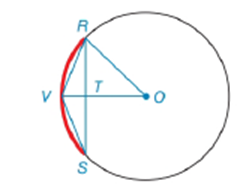Chapter 6.3, Problem 4EElementary Geometry For College St...

7th Edition
Alexander + 2 others
ISBN: 9781337614085

Solutions

Chapter
SectionElementary Geometry For College St...

7th Edition
Alexander + 2 others
ISBN: 9781337614085
Textbook Problem

Note: Exercises preceded by an asterisk are of a more challenging nature. G i v e n :                   V   i s   t h e   m i d p o i n t   o f     R S     i n     ⊙ O                                               m ∠ s = 15 ∘     a n d       O T = 6 F i n d :                     O RTo determine

To find:

To find OR.

Explanation

Given that V is the midpoint of RS^ in O and ms=15 and OT=6.

Consider the following figure,

Given that V is the midpoint of RS^ in O and ms=15.

Then mS=mRV^2 and mR=mRV^2. Then RS because these angles have equal measures.

Therefore,

15°=mRV^2

mRV^=2×15°

mRV^=30°

Therefore, mROV=mRV^=30°

Also OV¯RS¯ then,

Still sussing out bartleby?

Check out a sample textbook solution.

See a sample solution

The Solution to Your Study Problems

Bartleby provides explanations to thousands of textbook problems written by our experts, many with advanced degrees!

Get Started

Graph the following functions. 10.

Mathematical Applications for the Management, Life, and Social Sciences

Evaluate the integral. 55dx

Calculus (MindTap Course List)

True or False If the series converges to a number s, then all rearrangements of also converge to s.

Study Guide for Stewart's Single Variable Calculus: Early Transcendentals, 8th